# NCERT Solutions for Class 7 Maths Chapter 8 Comparing Quantities| PDF Download

Here you will find Chapter 8 Comparing Quantities NCERT Solutions for Class 7 Maths that will be helpful in improving the marks in the examinations and have edge over your classmates. You can download PDF of NCERT Solutions for Class 7 Maths Chapter 8 Comparing Quantities from this page through which one can able to solve the difficult problems given in a exercise. These NCERT Solutions are helpful resources that can help you not only cover the entire syllabus but also provide in depth analysis of the topics.

Class 7 NCERT Solutions are precious resources which can be used to figure out the latest marking scheme and improve the learning behaviour. It can be used to enrich knowledge and make lessons for learners more exciting.Exercise 8.1

1. Find the ratio of:
(a) Rs5 to 50 paise
(b) 15 kg to 210 g
(c) 9 m to 27 cm
(d) 30 days to 36 hours

To find ratios, both quantities should be in same unit.

(a) Rs 5 to 50 paise
⇒ 5 x 100 paise to 50 paise [∴ Rs 1 = 100 paise]
⇒ 500 paise to 50 paise
Thus, the ratio is = 500/50 = 10/1 = 10 : 1

(b) 15 kg to 210 g
⇒ 15 x 1000 g to 210 g [∵ 1kg = 1000 g]
⇒ 15000 g to 210 g
Thus, the ratio is = 15000/210 = 500/7 = 500:7

(c) 9 m to 27 cm
⇒ 9 x 100 cm to 27 cm [∵ 1m = 100 cm]
⇒ 900 cm to 27 cm
Thus, the ratio is = 900/27 = 100/3 = 100:3

(d) 30 days to 36 hours
⇒ 30 x 24 hours to 36 hours [∵ 1 day = 24 hours]
⇒ 720 hours to 36 hours
Thus, the ratio is = 720/36 = 20/1 = 20:1

2. In a computer lab, there are 3 computers for every 6 students. How many computers will be needed for 24 students?

∵ 6 students need = 3 computers
∵ 1 student needs = 3/6 computers
∵ 24 students need =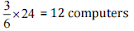Thus, 12 computers will be needed for 24 students.

3. Population of Rajasthan = 570 lakhs and population of U.P. = 1660 lakhs. Area of Rajasthan = 3 lakh km2 and area of U.P. = 2 lakh km2
(i) How many people are there per km2 in both states?
(ii) Which state is less populated?

(i) People present per km2 = Population/Area
In Rajasthan = 570 lakhs/3 lakhs per km2 = 190 people km2
In UP = 1660 lakhs/2 lakhs per km2 = 830 people per km2

(ii) Rajasthan is less populated.

Exercise 8.2

1. Convert the given fractional numbers to percent:
(a) 1/8
(b) 5/4
(c) 3/40
(d) 2/72. Convert the given decimal fractions to per cents:
(a) 0.65
(b) 2.1
(c) 0.02
(d) 12.353. Estimate what part of the figures is coloured and hence find the percent which is coloured.(i) Coloured part = 1/4
∴ Percent of coloured part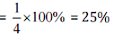(ii) Coloured part = 3/5
∴ Percent of coloured part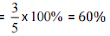(iii) Coloured part = 3/8
∴ Percent of coloured part= 37.5%

4. Find:
(a) 15% of 250
(b) 1% of 1 hour
(c) 20% of 2500
(d) 75% of 1 kg

(a) 15% of 250(b) 1% of 1 hours = 1% of 60 minutes = 1% of (60 x 60] seconds(c) 20% of Rs 5005. Find the whole quantity if:
(a) 5% of it is 600
(b) 12% of it is ₹1080
(c) 40% of it is 500 km
(d) 70% of it is 14 minutes
(e) 8% of it is 40 litres

Let the whole quantity be x in given questions:6. Convert given per cents to decimal fractions and also to fractions in simplest forms:
(a) 25%
(b) 150%
(c) 20%
(d) 5%

 S. No. Percents Fractions Simplest form Decimal form (a) 25% 25/100 1/4 0.25 (b) 150% 150/100 3/2 1.5 (c) 20% 20/100 1/5 0.2 (d) 5% 5/100 1/20 0.05

7. In a city, 30% are females, 40% are males and remaining are children. What percent are children?

Given: Percentage of females =30%
Percentage of males = 40%
Total percentage of females and males = 30 + 40 = 70%
Percentage of children = Total percentage - Percentage of males and females
= 100%-70%
= 30%
Hence, 30% are children.

8. Out of 15,000 voters in a constituency, 60% voted. Find the percentage of voters who did not vote. Can you now find how many actually did not vote?

Total voters = 15,000
Percentage of voted candidates = 60%
Percentage of not voted candidates = 100 - 60 = 40%
Actual candidates, who did not vote = 40% of 15000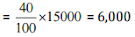Hence, 6,000 candidates did not vote.

9. Meeta saves Rs 400 from her salary. If this is 10% of her salary. What is her salary?

Let Meera's salary be Rs x.
Now, 10% of salary = Rs 400
⇒ 10% of x = Rs 400
Hence, Meera's salary is Rs 4,000.

10. A local cricket team played 20 matches in one season. It won 25% of them. How many matches did they win?

Number of matches played by cricket team = 20
Percentage of won matches = 25%
Total matches won by them = 25% of 20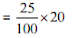= 5
Hence, they won 5 matches.

Exercise 8.3

1. Tell what is the profit or loss in the following transactions. Also find profit percent or loss percent in each case.
(a) Gardening shears bought for Rs 250 and sold for Rs 325.
(b) A refrigerator bought Rs12,000 and sold at Rs 13,500.
(c) A cupboard bought for Rs 2,500 and sold at Rs 3,000.
(d) A skirt bought for Rs 250 and sold at Rs 150.

(a) Cost price of gardening shears = Rs 250
Selling price of gardening shears = Rs 325
Since, S.P. > C.P., therefore here is profit.
∴ Profit = S.P. - C.P. = Rs 325 - Rs 250 = Rs 75
Now,(b) Cost price of refrigerator = Rs 12,000
Selling price of refrigerator = Rs 13,500
Since, S.P. > C.P., therefore here is profit.
∴ Profit = S.P. - C.P. = Rs 13500 - Rs 12000 = Rs 1,500
Now,Therefore, Profit = Rs 1,500 and Profit% = 12.5%

(c) Cost price of cupboard = Rs 2,500
Selling price of cupboard = Rs 3,000
Since, S.P. > C.P., therefore here is profit.
∴ Profit = S.P. - CP. = Rs 3,000 - Rs 2,500 = Rs 500
Now,Therefore, Profit = Rs 500 and Profit% = 20%

(d) Cost price of skirt = Rs 250
Selling price of skirt = Rs 150
Since, C.P. > S.P., therefore here is loss.
∴ Loss = CP, - S.P. = Rs 250 - Rs 150 = Rs100
Now,Therefore, Profit = Rs 100 and Profit% = 40%

2. Convert each part of the ratio to percentage:
(a) 3 : 1
(b) 2 : 3 : 5
(c) 1 : 4
(d) 1 : 2 : 5

(a) 3 :1
Total part =3 + 1= 4
Therefore, Fractional part = 3/4 : 1/4
⇒ Percentage of parts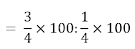⇒ Percentage of parts = 75% : 25%

(b) 2 : 3 : 5
Total part = 2 + 3 + 5 = 10
Therefore, Fractional part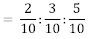⇒ Percentage of parts⇒ Percentage of parts = 20% : 30% : 50%

(c) 1:4
Total part =1 + 4 = 5
Therefore, Fractional part = 1/5 : 4/5
⇒ Percentage of parts⇒ Percentage of parts = 20%: 00%

(d) 1 : 2 : 5
Total part = 1+ 2 + 5 = 8
Therefore, Fractional part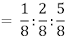⇒ Percentage of parts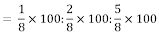⇒ Percentage of parts = 12.5% : 25% : 62.5%

3. The population of a city decreased from 25,000 to 24,500. Find the percentage decrease.

The decreased population of a city from 25,000 to 24,500.
Population decreased = 25,000 - 24,500 = 500
Decreased Percentage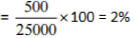Hence, the percentage decreased is 2%.

4. Arun bought a car for Rs 3,50,000. The next year, the price went up to Rs 3,70,000. What was the percentage of price increase?

Increased in price of a car from Rs 3,50,000 to Rs 3,70,000.
Amount change = Rs 3,70,000 - Rs 3,50,000 = Rs 20,000.
Therefore, Increased percentage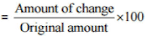Hence, the percentage of price increased is5. I buy a T.V. for Rs 10,000 and sell it at a profit of 20%. How much money do I get for it?

The cost price of T.V. = Rs 10,000
Profit percent = 20% Now,
Profit = Profit% of C.P,Selling price = C.P. + Profit = Rs 10,000 + Rs 2,000 = Rs 12,000
Hence, he gets Rs 12,000 on selling his T.V.

6. Juhi sells a washing machine for Rs 13,500. She loses 20% in the bargain. What was the price at which she bought it?

Selling price of washing machine = Rs. 13,500
Loss percent = 20%
Let the cost price of washing machine be Rs x.
Since, Loss = Loss% of C.P.
⇒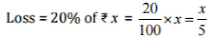Therefore, S.P. = C.P, - Loss7. (i) Chalk contains Calcium, Carbon and Oxygen in the ratio 10:3:12. Find the percentage of Carbon in chalk.
(ii) If in a stick of chalk, Carbon is 3 g, what is the weight of the chalk stick?

(i) Given ratio = 10 : 3 : 12
Total part = 10 + 3 + 12 = 25
Part of Carbon = 3/25
Percentage of Carbon part in chalk(ii) Quantity of Carbon in chalk stick = 3 g
Let the weight of chalk be x g.
Then, 12 % of x = 3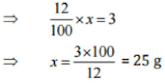Hence, the weight of chalk stick is 25 g.

8. Amina buys a book for Rs 275 and sells it at a loss of 15%. How much does she sell it for?

The cost of a book = Rs 275
Loss percent = 15%
Loss = Loss% of C.P. = 1 5 % of Rs 275Therefore, S.P. = C.P. - Loss = Rs 275 - Rs 41.25 = Rs 233.75
Hence, Amina sells a book for Rs 233.75.

9. Find the amount to be paid at the end of 3 years in each case:
(a) Principal = Rs 1,200 at 12% p.a.
(b) Principal = Rs 7,500 at 5% p.a.

(a) Here, Principal (P) = Rs 1,200, Rate (R) = 12% p.a., Time (T) = 3 yearsNow, Amount = Principal + Simple Interest
= Rs 1200 + Rs 432
= Rs 1,632
(b) Here, Principal (P) = Rs 7,500, Rate (R) = 5% p.a., Time (T) = 3 yearsNow, Amount = Principal + Simple Interest
= Rs 7,500 + Rs 1,125
= Rs 8,625

10. What rate gives Rs 280 as interest on a sum of Rs 56,000 in 2 years?

Here, Principal (P) = Rs 56,000, Simple Interest (S.I.) = Rs 280, Time (T) = 2 yearsHence, the rate of interest on sum is 0.25%.

11. If Meena gives an interest of Rs 45 for one year at 9% rate p.a. What is the sum she has borrowed?

Simple Interest = Rs 45, Rate (R) = 9% p.a., Time (T) = 1 years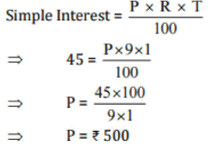Hence, she borrowed Rs 500.

## NCERT Solutions for Class 7 Maths Chapter 8 Comparing Quantities

Chapter 8 NERT Solutions is very useful in analyzing the problems and answering it with precision and the right concepts. Two ratios can be compared by converting them to like fractions. If the two fractions are equal, we say the two given ratios are equivalent.

• Ratio and proportion problems can be solved by using two methods, the unitary method and equating the ratios to make proportions, and then solving the equation.

• Percentage is another method used to compare quantities. Percentages are numerators of fractions with the denominator 100.

If you want exerciswise NCERT Solutions then you can get them just by clicking below. These NCERT Solutions for Class 7 will help you in understanding the important points inside the chapter properly.

Studyrankers experts have prepared these NCERT Solutions which will inculcate correct learning habits among students. A student will be able to get a deeper understanding of various topics.

### NCERT Solutions for Class 7 Maths Chapters:

 Chapter 1 Integers Chapter 2 Fractions and Decimals Chapter 3 Data Handling Chapter 4 Simple Equations Chapter 5 Lines and Angles Chapter 6 The Triangle and its Properties Chapter 7 Congruence of Triangles Chapter 9 Rational Numbers Chapter 10 Practical Geometry Chapter 11 Perimeter and Area Chapter 12 Algebraic Expressions Chapter 13 Exponents and Powers Chapter 14 Symmetry Chapter 15 Visualising Solid Shapes

FAQ on Chapter 8 Comparing Quantities

#### How many exercises are there in Chapter 8 Comparing Quantities Class 7 Maths NCERT Solutions?

There are three exercises in NCERT Solutions of Chapter 8 through which one can set a good foundation for future goals. These NCERT Solutions will guide students in a better way and make them confident.

#### How to convert percent into ratio?

Write the given per cent. Convert the given per cent into a fraction by dividing it by 100 and removing percent sign; %. Express the fraction obtained in step 2 in the simplest (or lowest) form. Express the fraction obtained in step 3 as a ratio.

#### How to convert ratio into percent?

Write the ratio; Let it be a : b. Convert the given ratio into the fraction a/b. Multiply the fraction as obtained as in step 2 by 100 and put per cent sign %.

#### How to convert decimal into percent?

To convert decimal into per cent, shift the decimal point two places to right and write % sign.